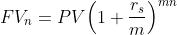Scan QR code or get instant email to install app

Question:

# An investor places $5,000 in an account. The stated annual interest rate is 6% compounded monthly. The value of the account at the end of three years is closest to: A$5,983.
explanation

1212With more than one compounding period per year,PV = 5,000; r = 6%; m = 12;;n = 3;mx n=12 x3 = 36.
To compute FV3, enter PV = -5,000; I = 0.5; PMT = 0; and N = 36 to get 5,983.40.